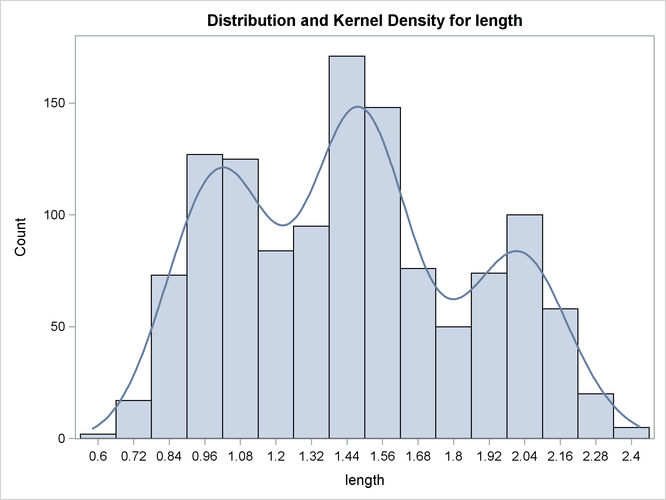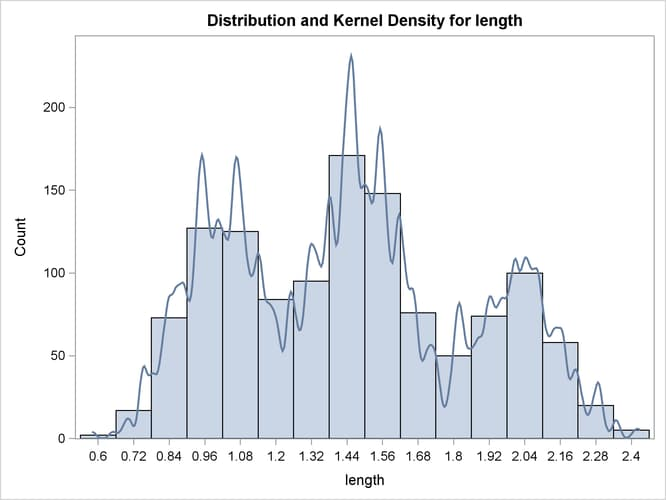The KDE Procedure

Example 48.2 Changing the Bandwidth

Continuing with Example 48.1, you can specify different bandwidth multipliers that determine the smoothness of the kernel density estimate. The following statements show kernel density estimates for the variable length by specifying two different bandwidth multipliers with the BWM= option:

proc kde data=channel;
univar length(bwm=2) length(bwm=0.25);
run;
ods graphics off;

Output 48.2.1 shows an oversmoothed estimate because the bandwidth multiplier is 2. Output 48.2.2 is created by specifying BWM=0.25, so it is an undersmoothed estimate.

Output 48.2.1: Histogram with Oversmoothed Kernel Density EstimateOutput 48.2.2: Histogram with Undersmoothed Kernel Density Estimate